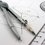Geometric Constructions.

This is a forum for construction problems. Rules:

• As the name says only geometry construction problems are allowed using a straightedge and a collapsible compass only/.
• A straightedge is an arbitrarily long straight edge, without any markings. You can connect two points, draw a line through a point or draw an arbitrary line using it.
• A collapsible compass is a circle drawing instrument which cannot be set to specific radii, but can draw a circle centred at a point passing through another, draw a circle centred at a point with an arbitrary radius or draw an arbitrary circle. However, it cannot copy a circle with the same radius after you draw one using it.
• All constructions must be completely defined. E.g. if you are asked to draw an inscribed rhombus, you must draw any sides that aren't already defined. You cannot leave it as 4 points which satisfy being the vertices of a rhombus.
• A move is defined as a use of either a straightedge or a collapsible compass. Marking in points doesn't count as a move.
• A construction can be done in moves if is the minimum number of moves required to create it.
• When you post solution of $n-$th problem you should post $n+1-$th problem(with number next to it...),then you get $3$ points otherwise you'll get $2$ point and moderator will post next one(problem).
• If one provides a different construction for any previous problem in the topic (even if it's earlier solved) which uses lesser number of moves then (s)he gets$3$ more points and $1$ point of the previous solver is deducted leaving the latter at $2$.
• After every problem I will sort the table(so that one with most point's is on top).
• If the $n-$th problem isn't solved for next $2$ days from the time it was posted then the poster should post new $n-$ th problem. But if someone solve first (version) of $n-$th problem it also counts. And yes must say, exercise your creativity in the limitless space of geometry. ~ XmL

Problem (Easy) 1: In a $\Delta ABC$, $T_B \in AC, T_C\in AB$ denotes the feet of the angle bisector corresponding to vertex $B$ and $C$, provide a construction as to restore the $\Delta ABC$ given the vertex $A$ and the feet of angle bisectors $T_B, T_C$.Note by The almighty Knows It All.
6 months, 2 weeks ago

This discussion board is a place to discuss our Daily Challenges and the math and science related to those challenges. Explanations are more than just a solution — they should explain the steps and thinking strategies that you used to obtain the solution. Comments should further the discussion of math and science.

When posting on Brilliant:

• Use the emojis to react to an explanation, whether you're congratulating a job well done , or just really confused .
• Ask specific questions about the challenge or the steps in somebody's explanation. Well-posed questions can add a lot to the discussion, but posting "I don't understand!" doesn't help anyone.
• Try to contribute something new to the discussion, whether it is an extension, generalization or other idea related to the challenge.
• Stay on topic — we're all here to learn more about math and science, not to hear about your favorite get-rich-quick scheme or current world events.

MarkdownAppears as
*italics* or _italics_ italics
**bold** or __bold__ bold

- bulleted
- list

• bulleted
• list

1. numbered
2. list

1. numbered
2. list
Note: you must add a full line of space before and after lists for them to show up correctly
paragraph 1

paragraph 2

paragraph 1

paragraph 2

> This is a quote
This is a quote
# I indented these lines
# 4 spaces, and now they show
# up as a code block.

print "hello world"
# I indented these lines
# 4 spaces, and now they show
# up as a code block.

print "hello world"
MathAppears as
Remember to wrap math in $$...$$ or $...$ to ensure proper formatting.
2 \times 3 $2 \times 3$
2^{34} $2^{34}$
a_{i-1} $a_{i-1}$
\frac{2}{3} $\frac{2}{3}$
\sqrt{2} $\sqrt{2}$
\sum_{i=1}^3 $\sum_{i=1}^3$
\sin \theta $\sin \theta$
\boxed{123} $\boxed{123}$

Sort by:

$\begin{array}{| l | l |}\hline \text{Name} &Score\\ \end{array}$

- 6 months, 2 weeks ago